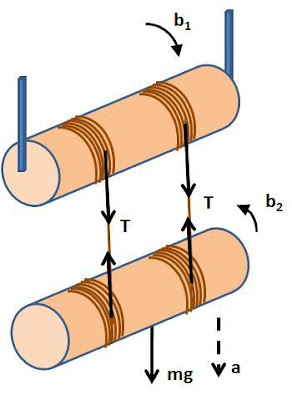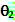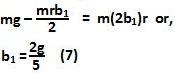## Sunday, December 14, 2008

### Irodov Problem 1.258Let the tension in the tension in each of the threads be T. This tension is responsible for inducing the spinning in both the cylinders. Let the downwards acceleration of the free cylinder be a. Let the radius of the cylinders be r. Also let the angular accelerations of the two cylinders be b1 and b2 as shown in the figure.

Spinning motion of the fixed cylinder: The net torque acting on the fixed cylinder is 2Tr. The moment of intertia of the cylinder is. So we have,Motion of the free cylinder: there are three forces acting on the free cylinder, i) the force of gravity mg and ii) the two tensions of value T in each of the threads. So we have,

The force of gravity passes through the axis of rotation of the free cylinder, so it does not induce any torque on the free cylinder. The two tensions however induce a torque of Tr each on the cylinder. So we have,From (1) and (3) it is clear the angular accelerations of both the cylinders are the same in magintude, in other words,

Figure above shows how various parts of the system move as the free cylinder descends. Consider that initially the point of contact of the thread and the fixed cylinder is A and that with the free cylinder is B. When the fixed cylinder spins an angle, thread of length ofis unwrapped from the fixed cylinder and so the point A descends by this length. Similarly when the free cylinder spins an angle, thread of lengthis unwrapped from the free cylinder. So the total length of string unwrapped is. The the total distance that the free cylinder descends is equal to the total length of thread unwrapped and is given by,

Using (1), (2) and (6) we have,From (7) and (1) we have,Anonymous said...

the sign of rotation b2 should be in opposite direction i.e. in anticlockwise direction.

Krishna Kant Chintalapudi said...

thanks for pointing out, i have corrected the direction in the pictureAnonymous said...

the equation jst abv eq(7) shld hv mrb1/2 on the left hand side...

Krishna Kant Chintalapudi said...

thanks, I have corrected the typo